Скачать презентацию SWAN 2006 Automation and Robotics Research Institute UTA

3f60b396bdae672364669e925d2ed39d.ppt

• Количество слайдов: 22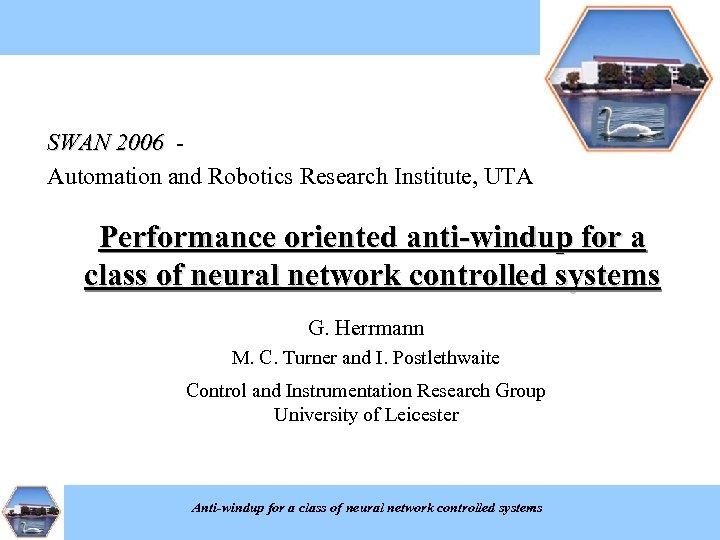SWAN 2006 Automation and Robotics Research Institute, UTA Performance oriented anti-windup for a class of neural network controlled systems G. Herrmann M. C. Turner and I. Postlethwaite Control and Instrumentation Research Group University of Leicester Anti-windup for a class of neural network controlled systems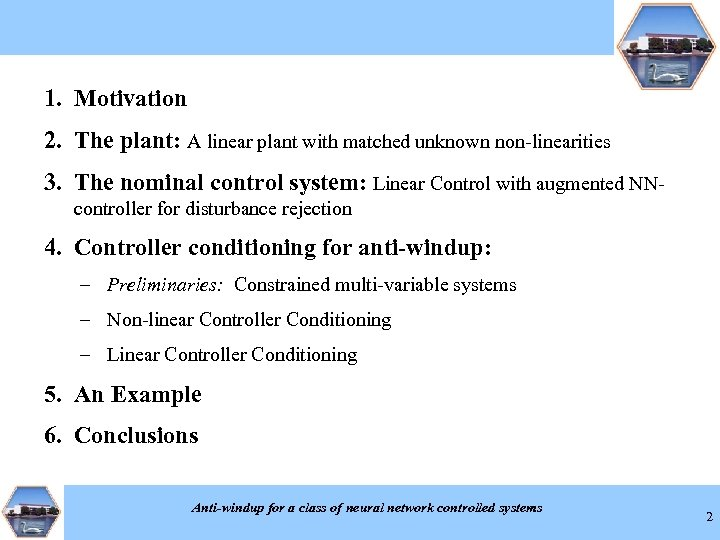1. Motivation 2. The plant: A linear plant with matched unknown non-linearities 3. The nominal control system: Linear Control with augmented NNcontroller for disturbance rejection 4. Controller conditioning for anti-windup: – Preliminaries: Constrained multi-variable systems – Non-linear Controller Conditioning – Linear Controller Conditioning 5. An Example 6. Conclusions Anti-windup for a class of neural network controlled systems 2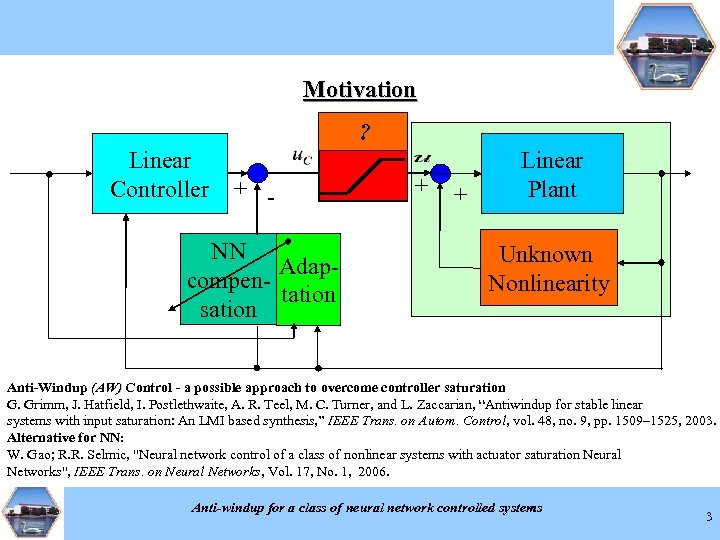Motivation ? Linear Controller + - NN Adapcompentation sation + + Linear Plant Unknown Nonlinearity Anti-Windup (AW) Control - a possible approach to overcome controller saturation of practical validation: Linear control performance in combination with NN-control – Examples NN-Control- Examples : G. Grimm, J. Hatfield, I. Postlethwaite, A. R. Teel, M. C. Turner, and L. Zaccarian, “Antiwindup for stable linear G. Herrmann, H. Lee, andand. J. Harris, Adaptive Neural Network Control a neural network controller in a hard S. S. Ge, C. G. Guo, “Practical implementation S. S. Ge, input saturation: An LMI based synthesis, ” IEEE Trans. onof of Robotic Manipulators. World 1509– 1525, 2003. systems with T. Autom. Control, vol. 48, no. 9, pp. Scientific, disk drive, ” IEEE Transactions on Control Systems Technology, 2005. Singapore, 1998. Alternative for NN: ——, Kim neural network controller Feedback to of high performance linear World Scientific, Y. “A and F. L. "Neural network control of a class a nonlinear systems with actuator saturation Neural W. Gao; R. R. Selmic, Lewis, High-Levelaugmented. Control with Neural Networks, "controller and its. Singapore, application to a Trans. on Neural Networks, Vol. system, ” IFAC 2005 (under journal review). 1998. Networks", IEEE HDD-track following servo 17, No. 1, 2006. Anti-windup for a class of neural network controlled systems 3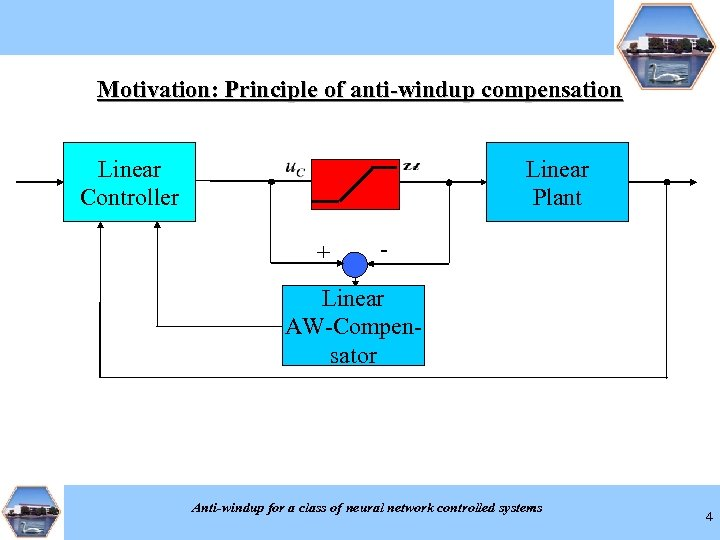Motivation: Principle of anti-windup compensation Linear Controller Linear Plant + - Linear AW-Compensator Anti-windup for a class of neural network controlled systems 4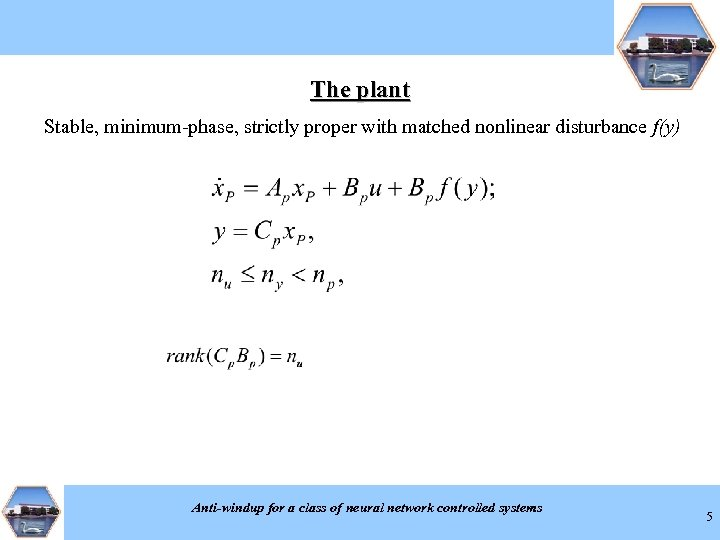The plant Stable, minimum-phase, strictly proper with matched nonlinear disturbance f(y) Anti-windup for a class of neural network controlled systems 5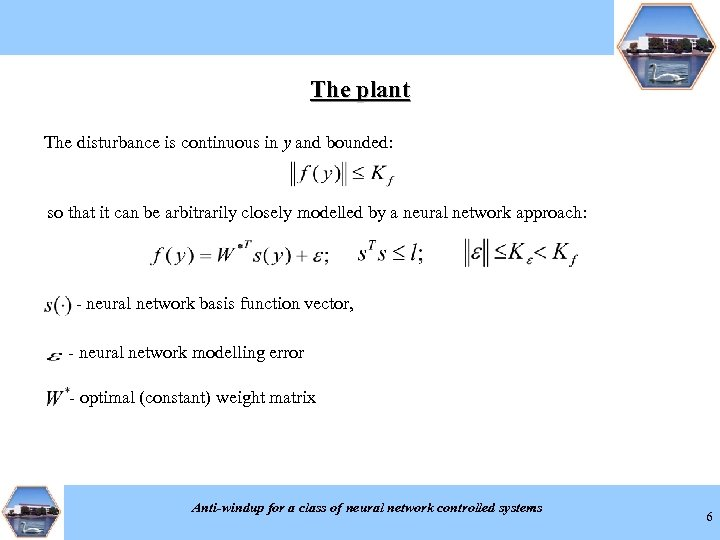The plant The disturbance is continuous in y and bounded: so that it can be arbitrarily closely modelled by a neural network approach: - neural network basis function vector, - neural network modelling error - optimal (constant) weight matrix Anti-windup for a class of neural network controlled systems 6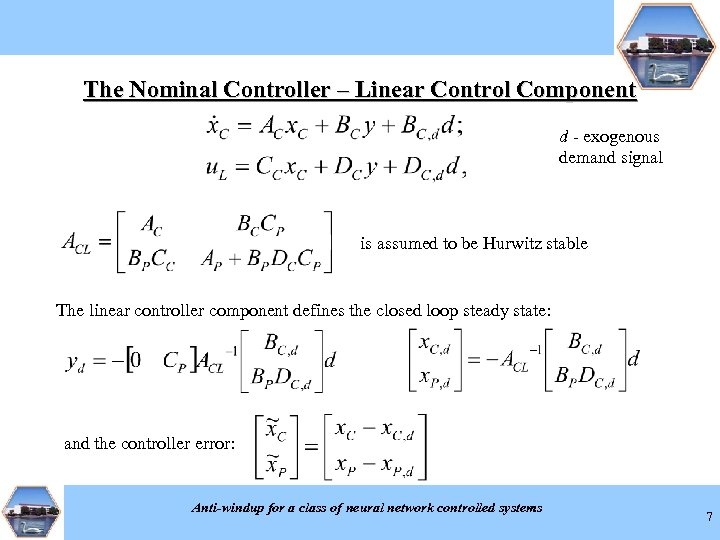The Nominal Controller – Linear Control Component d - exogenous demand signal is assumed to be Hurwitz stable The linear controller component defines the closed loop steady state: and the controller error: Anti-windup for a class of neural network controlled systems 7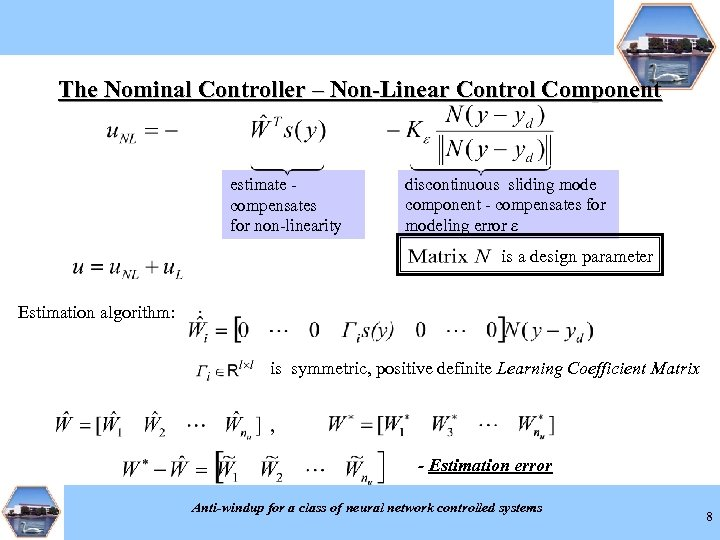The Nominal Controller – Non-Linear Control Component estimate compensates for non-linearity discontinuous sliding mode component - compensates for modeling error e is a design parameter Estimation algorithm: is symmetric, positive definite Learning Coefficient Matrix - Estimation error Anti-windup for a class of neural network controlled systems 8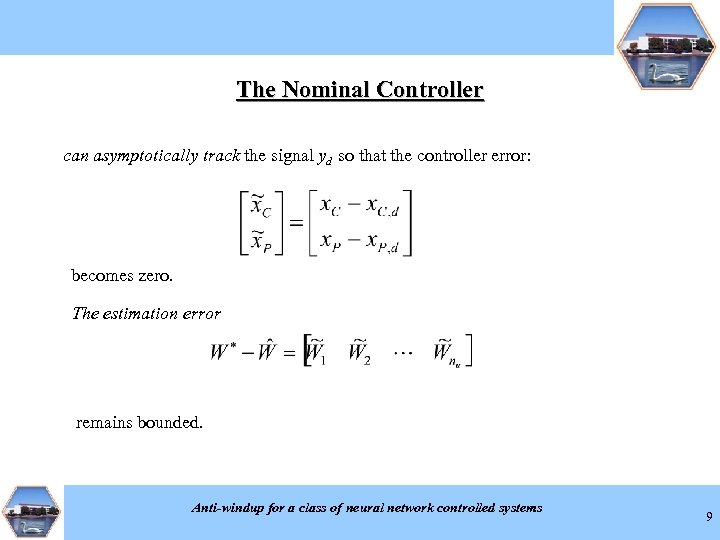The Nominal Controller can asymptotically track the signal yd so that the controller error: becomes zero. The estimation error remains bounded. Anti-windup for a class of neural network controlled systems 9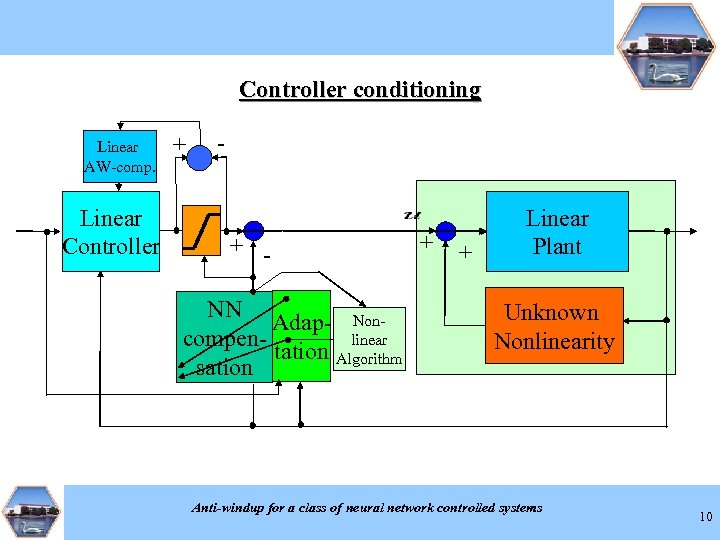Controller conditioning Linear AW-comp. Linear Controller + - + NN Adap- Nonlinear compentation Algorithm sation + + Linear Plant Unknown Nonlinearity Anti-windup for a class of neural network controlled systems 10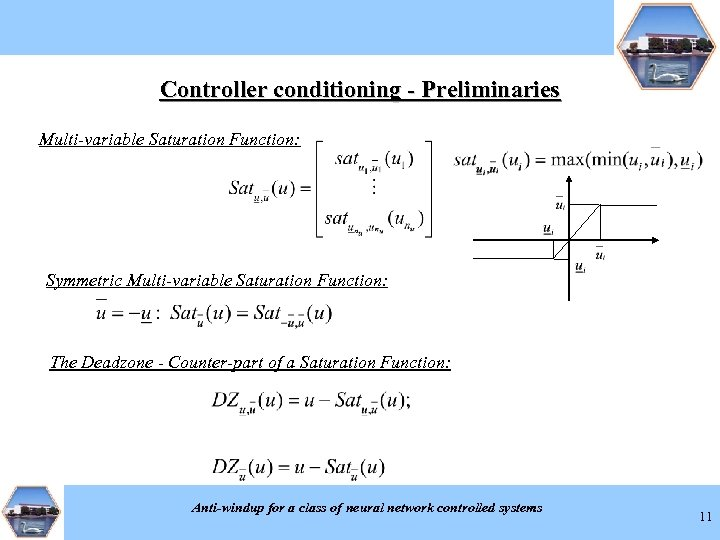Controller conditioning - Preliminaries Multi-variable Saturation Function: Symmetric Multi-variable Saturation Function: The Deadzone - Counter-part of a Saturation Function: Anti-windup for a class of neural network controlled systems 11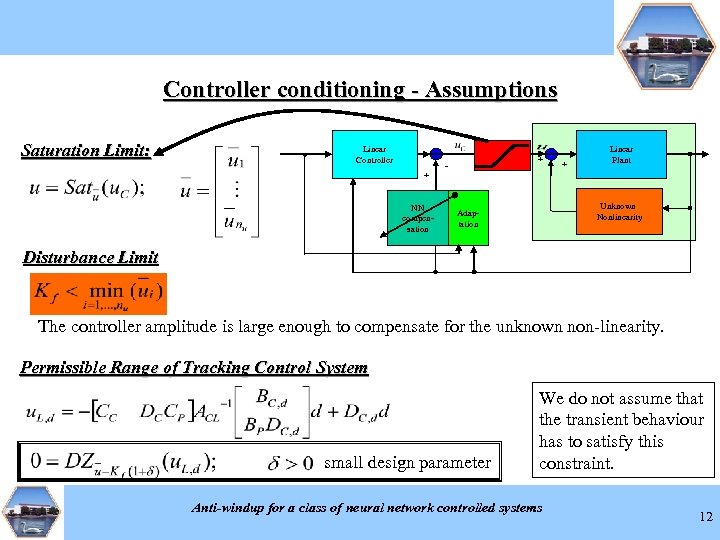Controller conditioning - Assumptions Saturation Limit: Linear Controller + NN compensation + - + Linear Plant Unknown Nonlinearity Adaptation Disturbance Limit The controller amplitude is large enough to compensate for the unknown non-linearity. Permissible Range of Tracking Control System small design parameter We do not assume that the transient behaviour has to satisfy this constraint. Anti-windup for a class of neural network controlled systems 12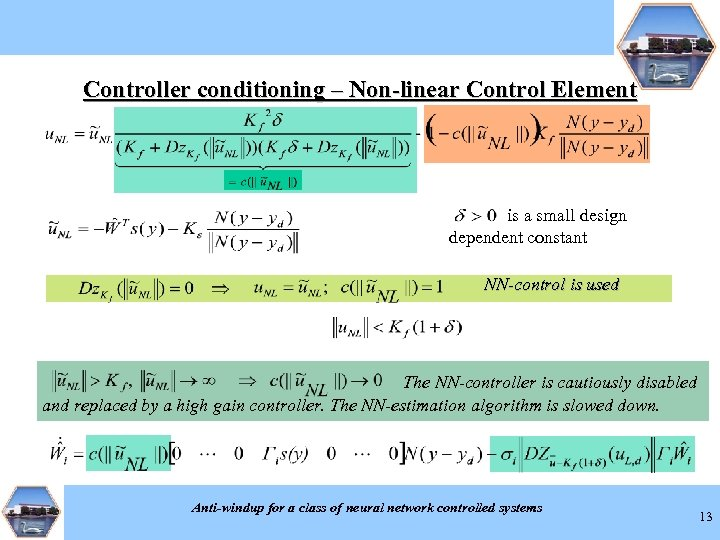Controller conditioning – Non-linear Control Element is a small design dependent constant NN-control is used The NN-controller is cautiously disabled and replaced by a high gain controller. The NN-estimation algorithm is slowed down. Anti-windup for a class of neural network controlled systems 13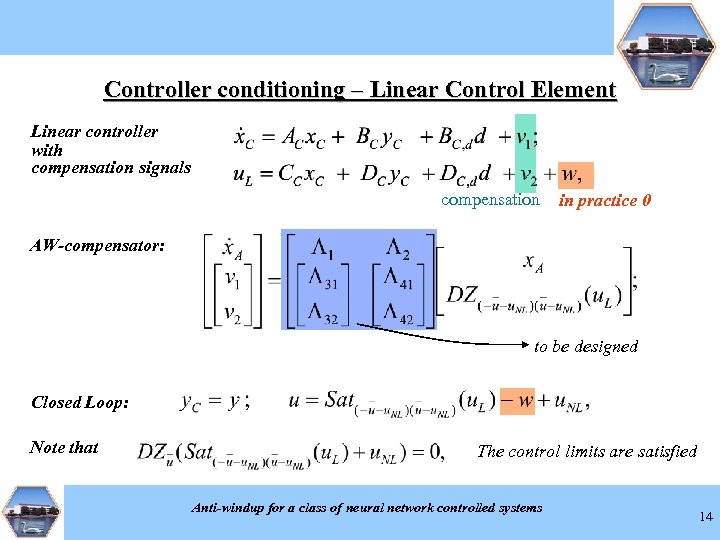Controller conditioning – Linear Control Element Linear controller with compensation signals compensation in practice 0 AW-compensator: to be designed Closed Loop: Note that The control limits are satisfied Anti-windup for a class of neural network controlled systems 14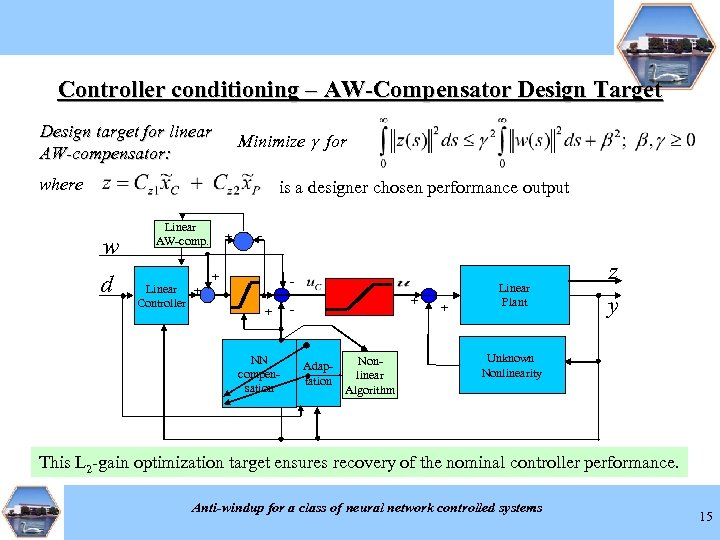Controller conditioning – AW-Compensator Design Target Design target for linear AW-compensator: Minimize g for where is a designer chosen performance output w d Linear AW-comp. Linear + Controller + - + + NN compensation + - Adaptation Nonlinear Algorithm + Linear Plant z y Unknown Nonlinearity This L 2 -gain optimization target ensures recovery of the nominal controller performance. Anti-windup for a class of neural network controlled systems 15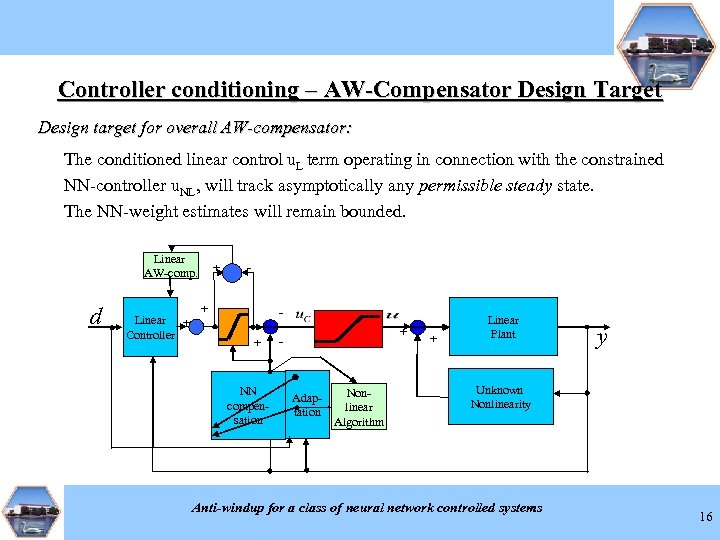Controller conditioning – AW-Compensator Design Target Design target for overall AW-compensator: The conditioned linear control u. L term operating in connection with the constrained NN-controller u. NL, will track asymptotically any permissible steady state. The NN-weight estimates will remain bounded. Linear AW-comp. d Linear + Controller + - + + NN compensation + - Adaptation Nonlinear Algorithm + Linear Plant y Unknown Nonlinearity Anti-windup for a class of neural network controlled systems 16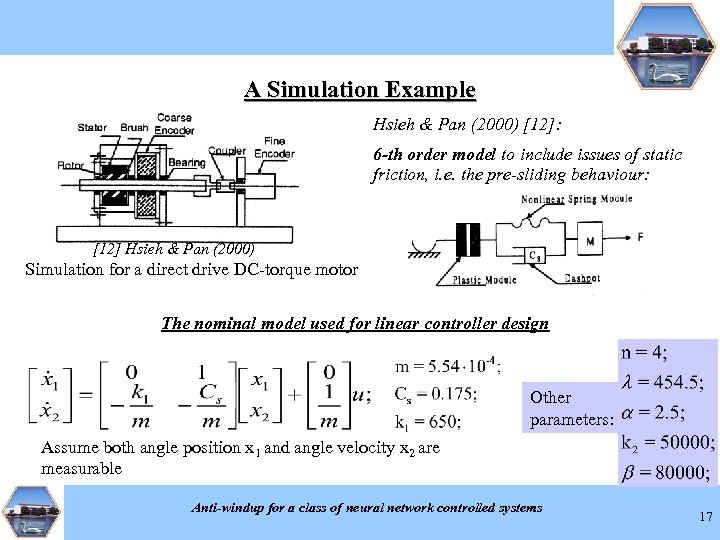A Simulation Example Hsieh & Pan (2000) : 6 -th order model to include issues of static friction, i. e. the pre-sliding behaviour:  Hsieh & Pan (2000) Simulation for a direct drive DC-torque motor The nominal model used for linear controller design Other parameters: Assume both angle position x 1 and angle velocity x 2 are measurable Anti-windup for a class of neural network controlled systems 17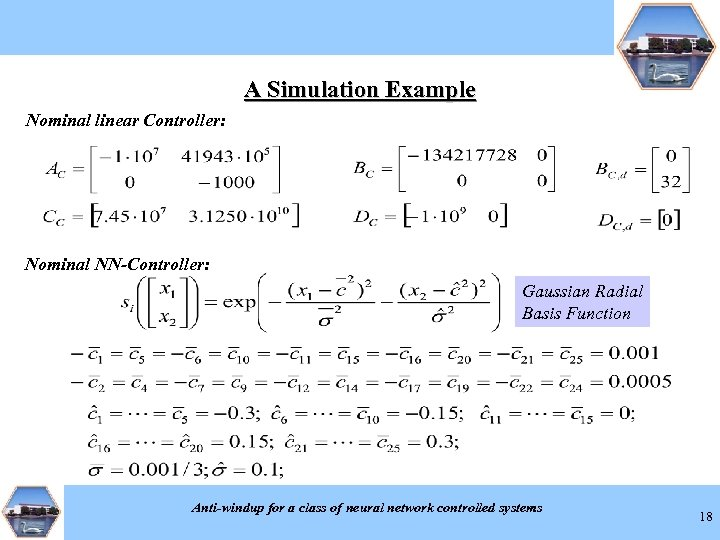A Simulation Example Nominal linear Controller: Nominal NN-Controller: Gaussian Radial Basis Function Anti-windup for a class of neural network controlled systems 18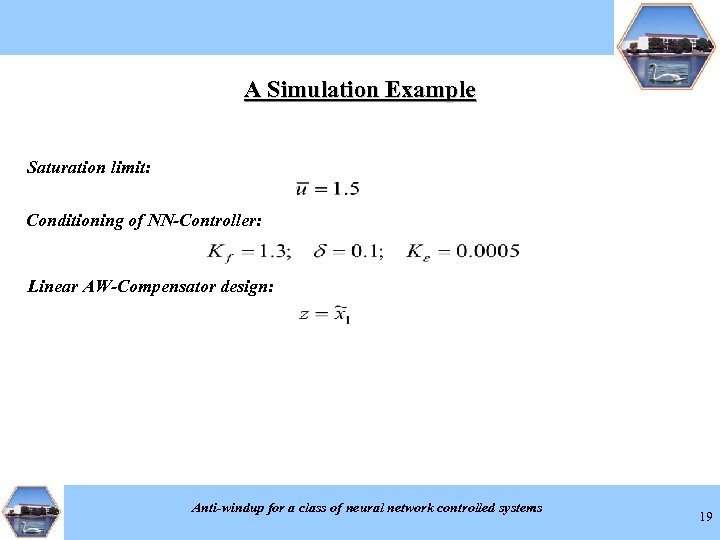A Simulation Example Saturation limit: Conditioning of NN-Controller: Linear AW-Compensator design: Anti-windup for a class of neural network controlled systems 19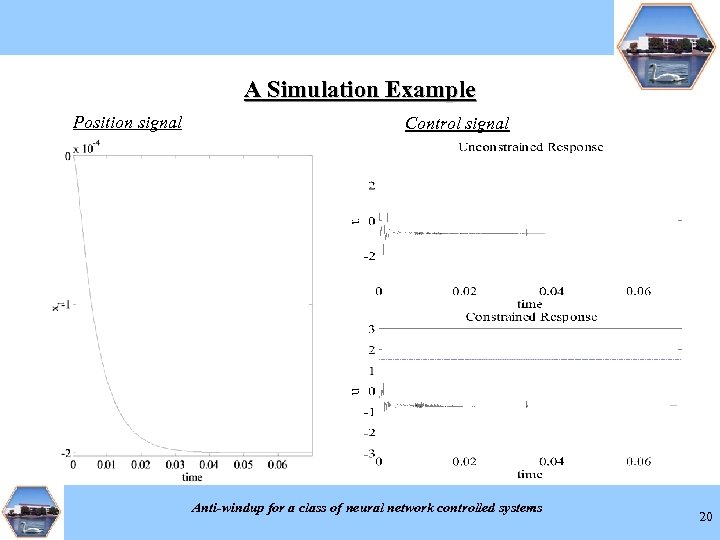A Simulation Example Position signal Control signal Anti-windup for a class of neural network controlled systems 20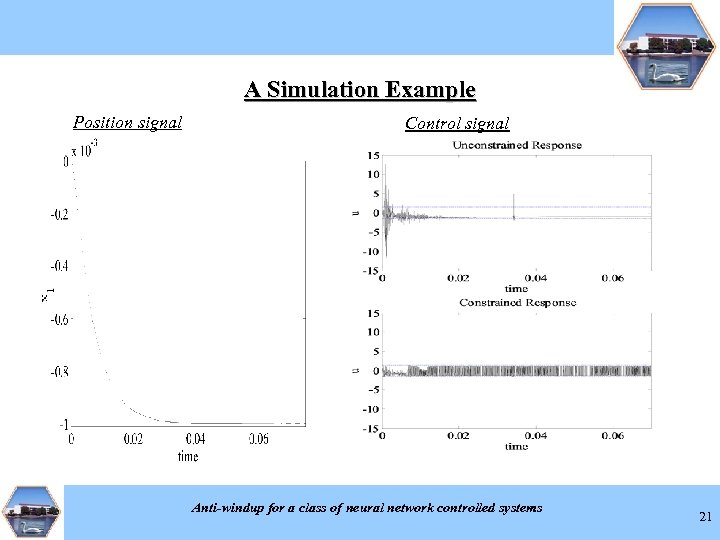A Simulation Example Position signal Control signal Anti-windup for a class of neural network controlled systems 21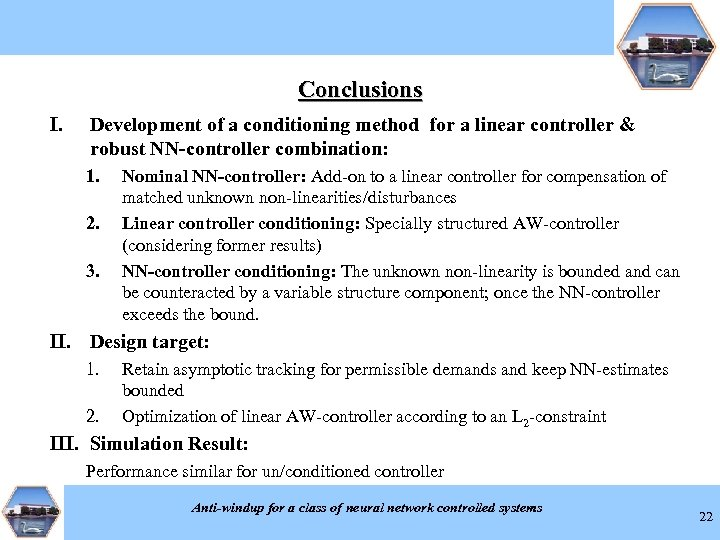Conclusions I. Development of a conditioning method for a linear controller & robust NN-controller combination: 1. 2. 3. Nominal NN-controller: Add-on to a linear controller for compensation of matched unknown non-linearities/disturbances Linear controller conditioning: Specially structured AW-controller (considering former results) NN-controller conditioning: The unknown non-linearity is bounded and can be counteracted by a variable structure component; once the NN-controller exceeds the bound. II. Design target: 1. 2. Retain asymptotic tracking for permissible demands and keep NN-estimates bounded Optimization of linear AW-controller according to an L 2 -constraint III. Simulation Result: Performance similar for un/conditioned controller Anti-windup for a class of neural network controlled systems 22